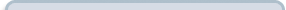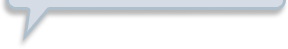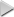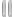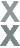# Prize Winner, Part 3

###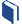Resources for this lesson: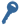Key Terms:

Experimental probability

You will use your Algebra II Journalon this page.

> Glossary> Calculator Resources> Teacher Resources: Instructional NotesCompare your simulation results (the experimental probability) with the theoretical probabilities.

##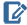Algebra II Journal: Reflection 2

Complete the chart shown below in your Algebra II Journal.

Prize

Probability to Win THEORETICAL

Probability to Win
EXPERIMENTAL

Small

$\frac{12}{27}$

Medium

$\frac{6}{27}$

Large

$\frac{1}{27}$

Out of your fifty (or more) trials, what is the probability that you win a small prize? Medium prize? Large prize? Record these probabilities in the chart you copied.

Respond to the following question in your Algebra II Journaland submit to your teacher.

• Compare the experimental probabilities with the theoretical probabilities. What conclusions can you draw?
• If you played this game, what prize would you try for? Use the results of your simulation to support your answer.